Graph Theory

Spanning Trees

Let G be a connected graph. A spanning tree in G is a subgraph of G that includes all the vertices of G and is also a tree. The edges of the trees are called branches.

For example, consider the following graph G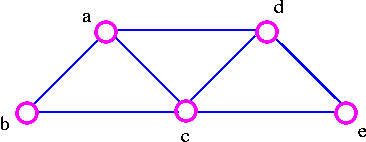The three spanning trees G are: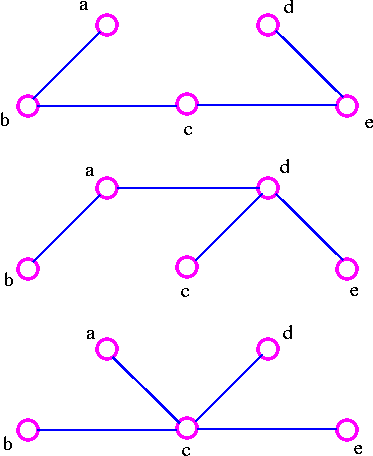We can find a spanning tree systematically by using either of two methods.

• Cutting-down Method
• Start choosing any cycle in G.
• Remove one of cycle's edges.
• Repeat this procedure until there are no cycle left.

For example, given the graph G1. We remove the edge ac which destroy the cycle adca in the above graph and we get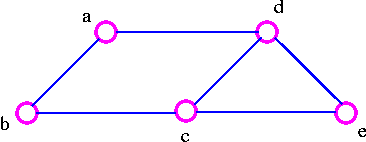2. We remove the edge cb, which destroy the cycle adcba in the above graph and we get3. We remove the edge ec, which destroy the cycle decd in the above graph and thus obtained the following spanning tree.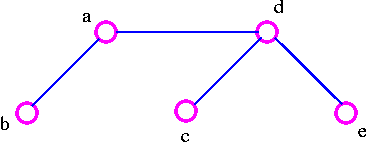• Building-up Method
• Select edges of G one at a time. in such a way that no cycles are created.
• Repeat this procedure until all vertices are included.

For example, for the following  graph G1. Choose the edge ab2. Next choose the edge de as follows: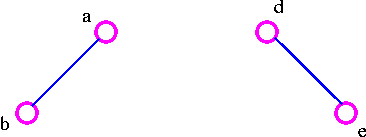3. After that choose the edge ec as follows: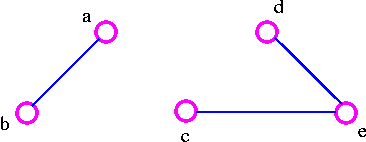4. Finally, we choose the edge cb and thus obtain the following spanning tree.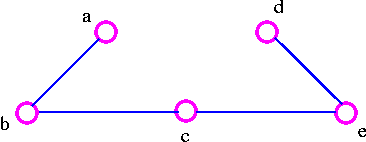Theorem     A graph is connected if and only if it has a spanning tree.

Proof    Let G be a connected graph. Delete edges from G that are not bridges until we get a connected subgraph H in which each edge is a bridge. Then H is a spanning tree. On the other hand, if there is a spanning tree in G, there is a path between any pair of vertices in G; thus G is connected.

Centers and Bicenters

It is convenient to start building up the tree at the middle of a tree and move outwards. This was the approach used by Arthur Cayley when he counted the number of chemical molecules by building them step by step. But what do we mean by the "middle" of a tree?

There are two straight forward ways to compute centers and bicenters.

Algorithm1

• Remove all the vertices of degree1, together with their incident edges.
• Repeat the process until we obtain either a single vertex (the center) or two vertices joined by an edge (the bicenter).

A tree with a center is called a central tree, and a tree with a bicenter is called a bicentral tree. Note that every tree is either central or bicentral, but not both.

For example, given a following tree.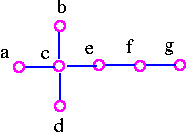Remove all vertices of degree 1.Remove all vertices of degree 1.Therefore, a tree is central with center e.

Another example, given a following tree.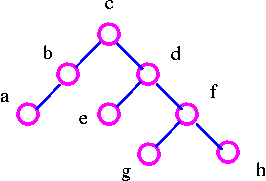Remove all vertices of degree 1.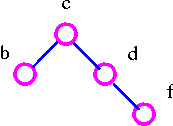Remove all vertices of degree 1.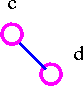Therefore, a given tree is bicentral with bicenter cd.

Algorithm 2

For each vertex v of the degree 2 or more, count the number of vertices in each of the subtrees emanating from v, and let nv be the maximum of these numbers. If the tree has n vertices it can be shown that either there is just one vertex v for which nv ≤ 1/2(n-1) (the centroid or centroid tree) or there are two adjacent vertices v and w for which nv = nw = 1/2n (the bicentroid or bicentroid tree). It is easy to see that every tree is either centroidal or bicentroidal, but not both.

Note that we can think of the centroid or bicentroid as the 'center of gravity' of the tree.

For example, given a following treeSince nc = 4, ne = 4, nf = 5 and ng = 6. Therefore, we have a bicentroidal tree with bicentroid ce.

Another example, given a following tree.Since nb = 6, nc = 5, nd = 3 and nf = 5. Therefore,  we have a centroidal tree with centroid d.